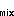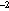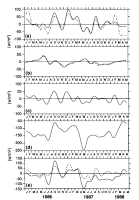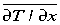U.S. Dept. of Commerce / NOAA / OAR / PMEL / Publications

## Variability of the sea surface temperature in the eastern equatorial Pacific during 1986-88

### (Mixed Layer Temperature Variability, continued)

#### Mixed Layer Heating

The moored temperature and velocity measurements on the equator (Figure 1) were interpolated, using a Laplacian interpolation scheme, to an evenly spaced grid from zero to 100 m with a resolution of 5 m. These gridded data were used in all subsequent calculations discussed here, and all derived quantities were low passed filtered with a 91-day Hanning filter prior to further analysis.

Figure 5 shows contour plots of the gridded and filtered temperature and velocity data. Superimposed on the temperature contours is the time series of mixed layer depth (h). This hwas estimated by finding the depth at which the contoured temperature was 0.5°C less than the SST. Linear interpolation was used between grid points. If the mixed layer was less than 5 m, it was set equal to 5 m. Note that the instrument resolution (Figure 1) restricts the accuracy of the depth determination to at best about ±5 m. The mixed layer was shallow in boreal spring and deepest in late summer or fall. On the seasonal time scale mixed layer depth and SST tend to be out of phase: warmest SST corresponds to a shallow mixed layer. However, the mixed layer in March 1987, during the ENSO warm event, was deeper than in either 1986 or 1988. The shallowest mixed layer occurred in spring 1988. Initially, from January to March 1988, this shoaling was associated with the seasonal warming; however, the mixed layer continued to be shallow as the SST dropped by nearly 8°C from March to May 1988. During this period the stratification extended to the surface and our approximation which assumes an isothermal surface layer is invalid.

The time rate of change of the mixed layer temperature (Q) was estimated from the data in Figure 5 using centered differences in time (Figure 6a, solid curve). The mixed layer heating assuming a constant layer thickness of 25 m (Figure 6a, dashed line) is also shown, since the estimate of mixed layer depth may be questionable given the vertical resolution of the instruments. The effect of the mixed layer depth variation was most important during January-February 1986 and January-May 1988, when the mixed layer was very shallow. During these periods, the amplitude of heating fluctuations computed from the variable mixed layer depth are considerably smaller than those obtained with a constant depth. In the latter period this difference amounted to nearly 60 W m. The constant mixed layer case indicated the rapid decrease in SST.Fig. 6. Time series plots of various terms in equation (1). All time series have been low pass filtered with a 91-day Hanning filter. ( a) Change in the mixed layer heat content, Q; the solid line was computed with variable h, and the dashed line has a constant h= 25 m. (b) Zonal advective heat flux, Q; the solid line was computed withT/x estimated from moorings at 110°W and 140°W, dashed line assumes a constant. (c) Vertical entrainment, Q. (d) Vertical diffusive heat flux, Q. (e) Superposition of Q(dashed line) and Q(solid line) as defined in the text.

Over the record length, the mean Q(using the variable h) was about 6 W mwith a standard deviation of 33 W m. Qualitative comparison of this mixed layer heating and net solar heat flux (Q, Figure 4) indicates large differences in the mean value and little correlation of the variability. The mean Qexceeds Qby more than 100 W mand the amplitude of Qfluctuations are too small to balance the changes in mixed layer heating. Evidently, the variability of mixed layer temperature must result from low-frequency imbalances of Qand the ocean processes included in equation (1). The terms in (1) which can be estimated from the long equatorial time series are discussed below.

Go back to previous section or forward to next section

PMEL Outstanding Papers

PMEL Publications Search

PMEL Homepage